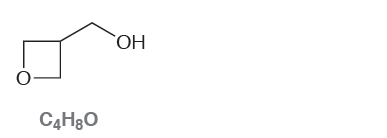# Problem: Consider the following compound with molecular formula  C 4H8O: (a) Draw a constitutional isomer that you expect will be  approximately one trillion (10 12) times more acidic than the compound above.

###### Problem Details

Consider the following compound with molecular formula  C 4H8O:

(a) Draw a constitutional isomer that you expect will be  approximately one trillion (10 12) times more acidic than the compound above.What scientific concept do you need to know in order to solve this problem?

Our tutors have indicated that to solve this problem you will need to apply the pKa concept. You can view video lessons to learn pKa. Or if you need more pKa practice, you can also practice pKa practice problems.

What is the difficulty of this problem?

Our tutors rated the difficulty ofConsider the following compound with molecular formula  C 4H...as high difficulty.

How long does this problem take to solve?

Our expert Organic tutor, Jonathan took 2 minutes undefined to solve this problem. You can follow their steps in the video explanation above.

What professor is this problem relevant for?

Based on our data, we think this problem is relevant for Professor Baldwin's class at OSU.

What textbook is this problem found in?

Our data indicates that this problem or a close variation was asked in Organic Chemistry - Klein 1st Edition. You can also practice Organic Chemistry - Klein 1st Edition practice problems.